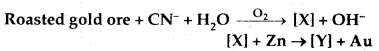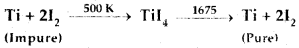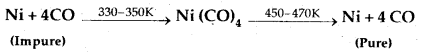# MCQ Questions for Class 12 Chemistry Chapter 6 General Principles and Processes of Isolation of Elements with Answers

We have compiled the NCERT MCQ Questions for Class 12 Chemistry Chapter 6 General Principles and Processes of Isolation of Elements with Answers Pdf free download covering the entire syllabus. Practice MCQ Questions for Class 12 Chemistry with Answers on a daily basis and score well in exams. Refer to the General Principles and Processes of Isolation of Elements Class 12 MCQs Questions with Answers here along with a detailed explanation.

## General Principles and Processes of Isolation of Elements Class 12 MCQs Questions with Answers

Question 1.
The salt which is least likely to be found in minerals is
(a) Chloride
(b) Sulphate
(c) Sulphide
(d) Nitrate

Question 2.
Which of the following metals is leached by Cyanide process?
(a) Ag
(b) Na
(c) Al
(d) Cu

Question 3.
In the equation 4 M + 8 CN + 2H2O + O2 → 4 [M(CN)2] + 4OH, the metal M is
(a) Copper
(b) Iron
(c) Gold
(d) Zinc

Question 4.
Pyrolusite is a/an
(a) Oxide ore
(b) Sulphide ore
(c) Carbide ore
(d) not an ore.

Question 5.
Which of the following metals is sometimes found native in nature?
(a) Aluminium
(b) Copper
(c) Iron
(d) Magnesium

Question 6.
In the process of extraction of gold.Identify the complexes [X] and [Y].
(a) X = [Au (CN)2], Y = [Zn (CN)4]2-
(b) X = [ Au (CN)4]3-, Y = [Zn (CN)4]2-
(c) X = [Au (CN)2], Y = [Zn (CN)6]4-
(d) X = [Au (CN)4], Y = [Zn(CN)4]2-

Answer: (a) X = [Au (CN)2], Y = [Zn (CN)4]2-

Question 7.
The method of zone refining of metals is based on the principle of
(a) greater mobility of pure metal than that of the impurity.
(b) higher m.pt. of the impurity than that of the pure metal.
(c) greater noble character of the solid metal than that of the impurity
(d) greater solubility of the impurity in the molten state than in the solid.

Answer: (d) greater solubility of the impurity in the molten state than in the solid.

Question 8.
In the extraction of copper from its sulphide ore, the metal is formed by the reduction of Cu2O with
(a) FeS
(b) CO
(c) Cu2S
(d) SO2.

Question 9.
Heating pyrites to remove sulphur is called
(a) Smelting
(b) Calcination
(c) Liquation
(d) roasting

Question 10.
In the metallurgy of which of the follo wing, cupellation process is used?
(a) Copper
(b) Silver
(c) Iron
(d) Aluminium

Question 11.
Which of the following metals is most abundant in the earth’s crust?
(a) Mg
(b) Na
(c) Al
(d) Fe

Question 12.
Which method of purification is represented by the equations:(a) Cupellation
(b) Poling
(c) van Arkel
(d) Zone-refining

Question 13.
Which of the following benefication process is used for the mineral Al2O3.2H2O?
(a) Froth Floatation
(b) Leaching
(c) Liquation
(d) Magnetic separation

Question 14.
Which method of purification is represented by the equations(a) vanArkel
(b) Vapour phase refusing
(c) Liquation
(d) Poling

Question 15.
The Mond’s process of refining is used for which of the following metals?
(a) Gold
(b) Copper
(c) Iron
(d) Nickel

Question 16.
During smelting an additional substance is added which combines with impurities to form a fusible mass. The additional substance is called
(a) Flux
(b) Slag
(c) Gangue
(d) Ore.

Question 17.
Which of the following metals is obtained by leaching the ore With dilute cyanide solution?
(a) Silver
(b) Titanium
(d) Zinc

Question 18.
Zone-refining process has been employed for preparing ultra pure samples of
(a) Cu
(b) Zn
(c) Ge
(d) Ag

Question 19.
The process of isolation of metals by dissolving the ore in a suitable chemical eagent following by precipitation of the metal by a more electropositive metal is called
(a) Electrometallurgy
(b) Hydrometallurgy
(c) Electro-refusing
(d) Zone-refining

Question 20.
Smelting involves reduction of the metal oxide with
(a) Carbon
(b) CO
(c) Magnesium
(d) Aluminum

Question 21.
Which of the following is an ore of Aluminium?
(a) Na3AlF6
(b) Al2O32H2O
(c) Al2O3
(d) Al2O3 H2O

Question 22.
The process of extracting the metal from its ore is called
(a) Metallurgy
(b) Refining
(c) Concentration
(d) Leaching

Question 23.
In the Froth floatation process for beneficiation of the ores, the ore particles float because
(a) they are light
(b) their surface is not easily wetted by water.
(c) they bear electrostatic charge
(d) they are insoluble.

Answer: (b) their surface is not easily wetted by water.

Question 24.
The process of concentrating Au and Ag ores is based upon their solubility in
(a) NH3
(b) HNO3
(c) HCl
(d) KCN

Question 25.
In the Aluminothermite process, aluminium acts as
(a) an oxidising agent
(b) a flux
(c) a reducing agent
(d) a solder